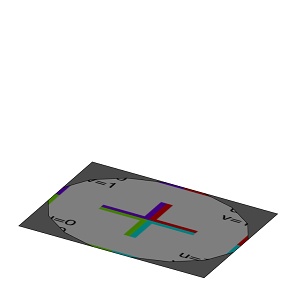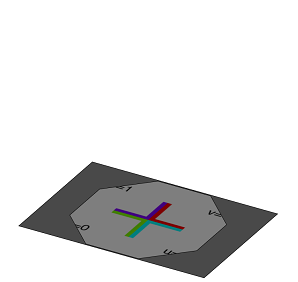# primitiveDisk

### Syntax

• primitiveDisk()
• primitiveDisk(nVertices)

### Parameters

1. nVerticesfloat
Number of vertices at the boundary of the disk. There must be at least 3 vertices. The default value is 16.

### Description

The primitiveDisk operation inserts a disk geometry into the scope of the current shape.

• If the radius isn’t given the bounding box coincides with the scope. Zero scope sizes are handled the same way as in the i operation.
• If radius is specified the disk is positioned in the xz-center of the scope lying on y=0.

If one scope size is zero while the 2 other sizes are not zero the disk will be inserted with respect to the plane defined by the valued scope sizes.

The disk has texture coordinates on the first texture layer (COLORMAP).

### Examples

 The inserted disk has 16 vertices and is fit into the current scope xy dimension. ``````Lot--> rotateScope(-90, 0, 0) // scope.sz is 0 primitiveDisk() texture("builtin:uvtest.png")``````The inserted disk has 8 vertices, a radius of 10 and is positioned in the xy center. ``````Lot--> rotateScope(-90, 0, 0) // scope.sz is 0 primitiveDisk(8,10) texture("builtin:uvtest.png")``````